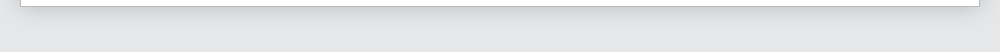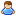Login Products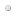SalesSupportDownloadsAbout
 Home » Technical Support » Elevate Web Builder Technical Support » Support Forums » Elevate Web Builder General » View Thread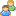View Thread

The following is the text of the current message along with any replies.
 Messages 1 to 5 of 5 total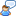Problem with Times
 Fri, Apr 27 2018 5:48 AM Permanent Link Paul CoshottHi All, I have been trying to work through a problem with times, when I noticed that a time of "12:05 AM" is saved and then comes back as "12:05 PM". So I tried the following: ShowMessage(TimeToStr(StrToTime('12:05 AM'))); This shows a message dialog with : "12:05 PM" This seems like a bug to me. Am I missing something? Cheers, Paul Fri, Apr 27 2018 10:55 AM Permanent Link Matthew Jones Paul Coshott wrote: > ShowMessage(TimeToStr(StrToTime('12:05 AM'))); > > This shows a message dialog with : "12:05 PM" > > This seems like a bug to me. Am I missing something? What is FormatSettings.ShortTimeFormat set to? I suspect it is ignoring the AM/PM. -- Matthew Jones Fri, Apr 27 2018 10:57 AM Permanent Link Walter Matte Tactical Business Corporation 00:05 or 00:05 AM works 13:05 AM returns 01:05 PM hmm... Walter Paul Coshott wrote: Hi All, I have been trying to work through a problem with times, when I noticed that a time of "12:05 AM" is saved and then comes back as "12:05 PM". So I tried the following: ShowMessage(TimeToStr(StrToTime('12:05 AM'))); This shows a message dialog with : "12:05 PM" This seems like a bug to me. Am I missing something? Cheers, Paul Fri, Apr 27 2018 4:12 PM Permanent Link Tim Young [Elevate Software] Elevate Software, Inc.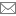timyoung@elevatesoft.com Paul, << I have been trying to work through a problem with times, when I noticed that a time of "12:05 AM" is saved and then comes back as "12:05 PM". >> It's a bug (actually two bugs), and here's the hot fixes: WebCore unit function TimeToStr(Value: DateTime; UTC: Boolean=False): String; var   I: Integer;   TempHour: Integer; begin   Result:='';   with FormatSettings do      begin      for I:=0 to 3 do         begin         case ShortTimeFormatComp[I] of            FULL_HOUR24_FORMAT:               Result:=Result+Pad(IntToStr(HourOf(Value,UTC)),2,'0');            PART_HOUR24_FORMAT:               Result:=Result+IntToStr(HourOf(Value,UTC));            FULL_HOUR12_FORMAT:               begin               TempHour:=HourOf(Value,UTC);               if (TempHour > AMPM_HOURS) then                  Dec(TempHour,AMPM_HOURS)               else if (TempHour=0) then <<<<<<<<<<<<<<<<                  Inc(TempHour,AMPM_HOURS); <<<<<<<<<<<<<<<<               Result:=Result+Pad(IntToStr(TempHour),2,'0');               end;            PART_HOUR12_FORMAT:               begin               TempHour:=HourOf(Value,UTC);               if (TempHour > AMPM_HOURS) then                  Dec(TempHour,AMPM_HOURS)               else if (TempHour=0) then  <<<<<<<<<<<<<<<<                  Inc(TempHour,AMPM_HOURS); <<<<<<<<<<<<<<<<               Result:=Result+IntToStr(TempHour);               end;            FULL_MIN_FORMAT:               Result:=Result+Pad(IntToStr(MinuteOf(Value,UTC)),2,'0');            PART_MIN_FORMAT:               Result:=Result+IntToStr(MinuteOf(Value,UTC));            FULL_SEC_FORMAT:               Result:=Result+Pad(IntToStr(SecondOf(Value,UTC)),2,'0');            PART_SEC_FORMAT:               Result:=Result+IntToStr(SecondOf(Value,UTC));            FULL_AMPM_FORMAT:               begin               TempHour:=HourOf(Value,UTC);               if (TempHour >= AMPM_HOURS) then                  Result:=Result+TimePMString               else                  Result:=Result+TimeAMString;               end;            end;         if (I < 3) then            begin            if (ShortTimeFormatComp[I+1] <> '') then               begin               if (ShortTimeFormatComp[I+1] <> FULL_AMPM_FORMAT) then                  Result:=Result+TimeSeparator               else                  Result:=Result+' ';               end;            end;         end;      end; end; function StrToTime(const Value: String; UTC: Boolean=False): DateTime; var   I: Integer;   TempComps: TStringsArray;   TempHour: Integer;   TempMinute: Integer;   TempSecond: Integer;   TempMessage: String; begin   Result:=DateTime(0);   TempMessage:=Translate('ERR_DATETIME_INVALID',[Translate('ERR_DATETIME_TIME'),Value]);   try      TempHour:=0;      TempMinute:=0;      TempSecond:=0;      with FormatSettings do         begin         TempComps:=ParseShortTime(Value);         for I:=0 to 3 do            begin            if (ShortTimeFormatComp[I]=FULL_HOUR24_FORMAT) or               (ShortTimeFormatComp[I]=PART_HOUR24_FORMAT) then               TempHour:=StrToInt(TempComps[I])            else if (ShortTimeFormatComp[I]=FULL_HOUR12_FORMAT) or                    (ShortTimeFormatComp[I]=PART_HOUR12_FORMAT) then               TempHour:=StrToInt(TempComps[I])            else if (ShortTimeFormatComp[I]=FULL_MIN_FORMAT) or                    (ShortTimeFormatComp[I]=PART_MIN_FORMAT) then               TempMinute:=StrToInt(TempComps[I])            else if (ShortTimeFormatComp[I]=FULL_SEC_FORMAT) or                    (ShortTimeFormatComp[I]=PART_SEC_FORMAT) then               TempSecond:=StrToInt(TempComps[I])            else if (ShortTimeFormatComp[I]=FULL_AMPM_FORMAT) then               begin               if SameText(TempComps[I],TimePMString) and (TempHour < AMPM_HOURS) then                  Inc(TempHour,AMPM_HOURS)               else if SameText(TempComps[I],TimeAMString) and (TempHour=AMPM_HOURS) then  <<<<<<<<<<<<<<<<                  Dec(TempHour,AMPM_HOURS);  <<<<<<<<<<<<<<<<               end;            end;         end;      if (not (IsHour(TempHour) and IsMinute(TempMinute) and IsSecond(TempSecond))) then         raise Exception.Create(TempMessage)      else         Result:=EncodeTime(TempHour,TempMinute,TempSecond,0,UTC);   except      raise Exception.Create(TempMessage);   end; end; Tim Young Elevate Software www.elevatesoft.com Sat, Apr 28 2018 2:49 AM Permanent Link Paul CoshottHi Tim, Thanks, working great now. Cheers, Paul